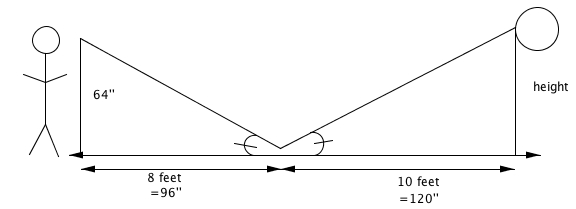### Home > CCG > Chapter Ch8 > Lesson 8.2.1 > Problem8-72

8-72.

Sandip noticed that when he looked into a mirror that was lying on the ground $8$ feet from him, he could see a clock on the wall. If Sandip’s eyes are $64$ inches off the ground, and if the mirror is $10$ feet from the wall, how high above the floor is the clock? Include a diagram in your solution.

Draw a diagram:Determine why the two triangles are similar. Remember triangles can be similar by AA, SAS, and SSS similarity theorems.

Set up a proportion. Are your units the same?

Solve the equation.